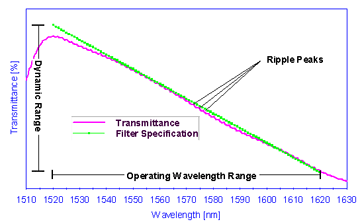# Linear Transmittance Filter Tutorial

Linear Transmittance Filter (LTF) has a transmittance that is changing linearly over a specified wavelength range. LTFs are typically used for wavelength identification applications. For this reason, it is critical that the linear filters be monotonic in transmittance over the wavelength range of interest as there must be a one-to-one correspondence between the filter transmittance and a given wavelength.

A Linear Transmittance Filter (LTF) linearly attenuates the transmittance over a broad range of wavelengths. The relationship between attenuation level and wavelength is linear such that the slope of the attenuation vs. wavelength is constant and monotonically increasing or decreasing.

LTFs are used for wavelength identification and wavelength drift monitoring. Wavelength identification applications use LTFs to determine the wavelength of a light signal based on the signal’s relative intensity. Changes in the signal’s intensity can be then be used to determine the wavelength drift.## Operating Wavelength Range:

The operating wavelength range is that which is defined by the specifications. Typically this range corresponds to the wavelengths at the extrema of the dynamic range.

## Dynamic Range:

The dynamic range is the difference between the maximum transmittance and minimum transmittance over the specified wavelength range.

## Monotonic:

A monotonic filter has the property that the transmittance is always increasing (or decreasing) transmittance over a specified wavelength range, i.e. the sign of the slope over this wavelength range does not change. This property is affected by the amount of ripple present.

## Substrate Etalon Ripple Effect [dB]:

With coherent light, any type of filter can act as a Fabry-Perot etalon if the two surfaces of the filter are sufficiently parallel and if the secondary surface of the filter is not anti-reflectance (AR) coated. If this is the case, then there will be an etalon ripple superimposed on the desired filter shape. For a substrate thickness of 1 mm, the resultant ripple will have a period of ~0.8 nm at a wavelength of 1550 nm. The magnitude of the ripple will depend on the reflectance of the two sides of the filter and the substrate parallelism. This etalon ripple can be minimized by introducing a sufficient wedge angle (> 0.2?) or by depositing a very good AR coating on the secondary surface.

## Slope:

The slope of the linear target is defined as the dynamic range of target divided by wavelength range.

#### Send Us an Enquiry

This field is for validation purposes and should be left unchanged.